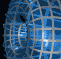Português | English Beyond 3D > In- and Outside the Torus > Mathematics

# The Mathematics of In- and Outside the Torus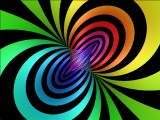Unlike the tori in "Torus Triptych", this is a projection of a torus lying originally in four-space, given parametrically by (cos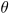, sin, cos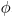, sin). Notice that this represents the cross product of two circles, one in the first two coordinates, and one in the second two. Note also that every point on this torus is at a distance of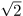from the origin, so the entire torus lies on the sphere of radiusin four-space. (In fact, this torus separates the sphere into two congruent solid tori.) Stereographic projection from the point (0, 0, 0,) in four-space yields an image of the torus in three-space, and since stereographic projection maps circles to circles, the image of the torus given above under this map would be a torus of revolution. In contrast to the parameterization given for the "Torus Triptych", however, this one has the interesting property that it is a conformal mapping of the ()-plane onto the torus of revolution.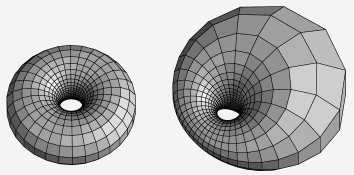A torus projected from four-space can look like a torus of revolutiuon (left). If part of it is closer to the projection point, part will appear larger, and it will form a cyclide of Dupin.

If we rotate the original torus in four-space before projecting it, the image changes: a portion of the torus moves closer to the point of projection, so its image gets larger (just as a shadow gets larger if you move an object closer to the light source), and part of it moves farther away from the point of projection, so its image gets smaller. In the projection of the torus, we would see one part of the ring get thicker and the opposite part get thinner. The result is known as a cyclide of Dupin. (The offset surfaces of these projections are also cyclides, and it turns out that every cyclide can be produced in this way.) As the torus rotates further, the torus gets fatter and fatter on one side, and thinner and thinner on the other. After a rotation of 90 degrees, the torus will pass through the point of projection, and so its image will appear to extend to infinity; the images of the two generating circles for the torus will be two infinite, straight lines in three-space. (Two other circles on the torus also map to straight lines in three-space: the (1,1)-curve, described below, that passes through the point of projection, and the analogous (1,-1)-curve.) As the torus rotates further, its image again becomes a finite torus, but what was outside the original torus is now inside, and vice versa; the torus in three-space has "turned inside out" by passing through infinity.

The image "In- and Outside the Torus" represents the 90 degree rotation, the point of transition when the outside and inside are begin exchanged. Indeed, at this instant, the image of the torus divides all of three-space into two congruent parts, the images of the two solid tori mentioned earlier that form the three-sphere in four-space with this torus as their common boundary. In the picture, the viewer is in one of the two congruent pieces (with the handle of the torus moving horizontally through the center of the picture), and the other piece is "behind" the surface (with the handle moving vertically through the center). A rotation of three-space about a diagonal line from the upper left to the lower right would interchange the two congruent pieces. In the picture, bands on the torus are removed to help make the structure clearer. In this case, the bands are formed by neighborhoods of the (1,1)-curves on the torus, which are the images of curves of the form=+ c in the ()-plane.

For those interested in producing similar pictures themselves, we describe the stereographic projection map and rotations in four-space in more detail. Stereographic projection from the point (0,0,0,d) is the map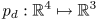given by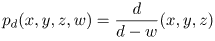for all points where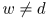. In our case, d =. As with rotations in the xy-plane, rotations in four-space can be represented by matrix multiplication. For example, a rotation in the xw-plane by an angle of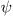is given by the map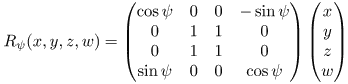The composition of these two maps with the parameterization of the torus given above will yield the series of pictures described here (asvaries from 0 to 90 degrees and beyond).